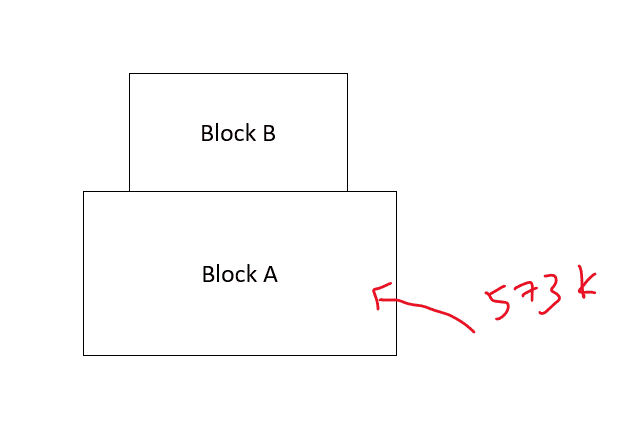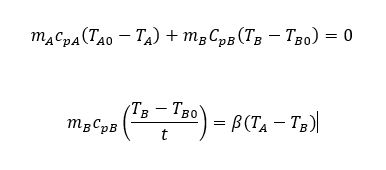# Heat transfer between objects by conduction

In summary, the model assumes that contact resistance is negligible, and solves for the time needed for block B to reach a particular temperature.

Hi guys, I'm a 2nd year mechanical student currently taking heat and mass transfer. A question came up in my mind when I was looking into the conduction heat transfer.

If I put block B on block A where block A is 573K and block B is 303K. Is there any way that I can calculate the time required for block B to be heated to a certain temperature let's say 398K?I understand that the conduction equation is Q/t = kA((T1-T2)/L). But I really wanted to know how can this be used to solve the problem above.
I would really appreciate it if anyone could help to clear my doubt.

Do you think the temperatures in the two blocks will be uniform spatially during the equilibration? Are you familiar with the transient heat conduction equation?

Try making some simplifying assumptions as a first model:

Blocks with very large thermal conductivities ##k##, and reasonably sized blocks should imply spatial thermal gradients to be negligible. What this says is that the Temperatures ##T_A, T_B## are uniformly distributed throughout each block at any time ##t##.

For simplicity, assume conservation of energy between the two blocks (the two blocks are the entire system). What that means is the heat absorbed by block B from block A, is exactly the heat lost from block A.

$$\cancel{ \Delta Q} = m_A c_{p_A} \Delta T_A + m_B c_{p_B} \Delta T_B = 0 \tag{1}$$

And for the dynamic portion try applying a surface energy balance at the interface of blocks A and B using a thermal contact resistance type model.

$$m_B c_{p_B} \frac{dT_B}{dt} = \beta \left( T_A - T_B \right) \tag{2}$$

## \beta## is a parameter that would depend on the area of contact and the pressure of contact.

Using ##(1)## and the initial conditions ##T_{A_o}, T_{B_o}## you can solve ##(2)## for the time ##t## needed for block B to reach a particular ##T_B##.

Note: The model above assumes ##T_A > T_B##

•erobz said:
Try making some simplifying assumptions as a first model:

Blocks with very large thermal conductivities ##k##, and reasonably sized blocks should imply spatial thermal gradients to be negligible. What this says is that the Temperatures ##T_A, T_B## are uniformly distributed throughout each block at any time ##t##.

For simplicity, assume conservation of energy between the two blocks (the two blocks are the entire system). What that means is the heat absorbed by block B from block A, is exactly the heat lost from block A.

$$\cancel{ \Delta Q} = m_A c_{p_A} \Delta T_A + m_B c_{p_B} \Delta T_B = 0 \tag{1}$$

And for the dynamic portion try applying a surface energy balance at the interface of blocks A and B using a thermal contact resistance type model.

$$m_B c_{p_B} \frac{dT_B}{dt} = \beta \left( T_A - T_B \right) \tag{2}$$

## \beta## is a parameter that would depend on the area of contact and the pressure of contact.

Using ##(1)## and the initial conditions ##T_{A_o}, T_{B_o}## you can solve ##(2)## for the time ##t## needed for block B to reach a particular ##T_B##.

Note: The model above assumes ##T_A > T_B##
What if there is negligible contact resistance? How would you handle the transient heat transfer calculation then?

Chestermiller said:
What if there is negligible contact resistance? How would you handle the transient heat transfer calculation then?
The blocks are in physical contact, there should always be some contact resistance. But, I don't know. I was just proposing the simplest model I could get to (solvable by someone at my level of education). What would you do?

erobz said:
The blocks are in physical contact, there should always be some contact resistance. But, I don't know. I was just proposing the simplest model I could get to (solvable by someone at my level of education). What would you do?
I would handle this by solving the transient heat conduction equation: $$\rho C_p\frac{\partial T}{\partial t}=k\left[\frac{\partial ^2 T}{\partial x^2}+\frac{\partial ^2 T}{\partial y^2}\right]$$If the two blocks are made of the different materials, the thermal properties would be different for each block. In addition, if they were made of different materials, the heat flux in the y-direction would be continuous at the interface: $$\left[k_A\frac{\partial T}{\partial y}\right]_A=\left[k_B\frac{\partial T}{\partial y}\right]_B$$The temperature gradient would be zero at all other boundaries of the blocks.

Last edited:
•Chestermiller said:
I would handle this by solving the transient heat conduction equation: $$\rho C_p\frac{\partial T}{\partial t}=k\left[\frac{\partial ^2 T}{\partial x^2}+\frac{\partial ^2 T}{\partial y^2}\right]$$If the two blocks are made of the different materials, the thermal properties would be different for each block. In addition, if they were made of different materials, the heat flux in the y-direction would be continuous at the interface: $$\left[k_A\frac{\partial T}{\partial y}\right]_A=\left[k_B\frac{\partial T}{\partial y}\right]_B$$The temperature gradient would be zero at all other boundaries of the blocks.
Using that, what would be the solution of minimum complexity that would adequately describe it?

Last edited:
erobz said:
Using that, what would be the solution of minimum complexity that would adequately describe it?
Numerical solution.

•Lord Jestocost
Chestermiller said:
Do you think the temperatures in the two blocks will be uniform spatially during the equilibration? Are you familiar with the transient heat conduction equation?
Hi Chestermiller, thanks for your reply, and sorry for the late response. I think the temperatures in the two blocks will not be uniform spatially during the equilibration, they vary when near their final temperatures. I'm not familiar with the transient heat conduction equation, but I will look into it!

erobz said:
Try making some simplifying assumptions as a first model:

Blocks with very large thermal conductivities ##k##, and reasonably sized blocks should imply spatial thermal gradients to be negligible. What this says is that the Temperatures ##T_A, T_B## are uniformly distributed throughout each block at any time ##t##.

For simplicity, assume conservation of energy between the two blocks (the two blocks are the entire system). What that means is the heat absorbed by block B from block A, is exactly the heat lost from block A.

$$\cancel{ \Delta Q} = m_A c_{p_A} \Delta T_A + m_B c_{p_B} \Delta T_B = 0 \tag{1}$$

And for the dynamic portion try applying a surface energy balance at the interface of blocks A and B using a thermal contact resistance type model.

$$m_B c_{p_B} \frac{dT_B}{dt} = \beta \left( T_A - T_B \right) \tag{2}$$

## \beta## is a parameter that would depend on the area of contact and the pressure of contact.

Using ##(1)## and the initial conditions ##T_{A_o}, T_{B_o}## you can solve ##(2)## for the time ##t## needed for block B to reach a particular ##T_B##.

Note: The model above assumes ##T_A > T_B##
Hi erobz, thank you for your reply and sorry for the late response. Your explanation is clear. I would like to ask so if I would like to use the equations that you provided above, It will be solving like this right?Hi erobz, thank you for your reply and sorry for the late response. Your explanation is clear. I would like to ask so if I would like to use the equations that you provided above, It will be solving like this right?
View attachment 313553
Close, but the second one is going to be an ODE ( linear - first order - non homogeneous). What you have written assumes the rate of change in temperature is constant in time.

Solve ##(1)## algebraically for ##T_A ## as a function of ##T_B##.

Then you plug that result into ##(2)## ( eliminating ##T_A## ) and solve the resulting ODE by standard methods.

Just as warning, what I've done is very idealized model. If you are looking for more realistic results you are going to waiting until after heat transfer/mass transfer course is nearly completed to revisit this problem IMO using the techniquies @Chestermiller has suggested.

Last edited:
erobz said:
Close, but the second one is going to be an ODE ( linear - first order - non homogeneous). What you have written assumes the rate of change in temperature is constant in time.

Solve ##(1)## algebraically for ##T_A ## as a function of ##T_B##.

Then you plug that result into ##(2)## ( eliminating ##T_A## ) and solve the resulting ODE by standard methods.

Just as warning, what I've done is very idealized model. If you are looking for more realistic results you are going to waiting until after heat transfer/mass transfer course is nearly completed to revisit this problem IMO using the techniquies @Chestermiller has suggested.
Alright, erobz thanks for correcting me! I understand that the rate of change in temperature is variable in time. But I'm curious and just want to ask if I assume the rate of change in temperature is constant in time, will I get any approximate result?

Alright, erobz thanks for correcting me! I understand that the rate of change in temperature is variable in time. But I'm curious and just want to ask if I assume the rate of change in temperature is constant in time, will I get any approximate result?
I doubt it. You probably won't even get an approximate result if you solve the ODE as it is. You could amend the system of equations to account for "real world" stray heat transfer to the environment from both blocks, but it's going to get more involved. I'm afraid in the end (as I think you will find out) heat transfer is messy business...that requires painstaking tedium to get half-truths at best. Thats my opinion of the subject, the experts probably disagree, but...

I'll share with you the solution I get with the model as is:

With

##\beta## a parameter with units ## \rm{ \frac{W}{m^2 K}}##
##\gamma = \frac{m_B c_B}{m_A c_A}##
##A_c## is the contact area
## T_B## is temp of the block at time ##t##

$$t = \frac{1}{\beta A_c} \frac{m_B c_B}{1+\gamma} \ln \left( \frac{T_{A_o} + \gamma T_{B_o} - \left( 1+ \gamma\right) T_{B_o} }{ T_{A_o} + \gamma T_{B_o} - \left( 1+ \gamma\right) T_B } \right)$$

This model is valid for block B temperatures ##T_B## in the range:

$$T_{B_o} \leq T_B \leq \frac{ T_{A_o} + \gamma T_{B_o} }{ 1+ \gamma}$$

You can see that there is going to be this parameter ##\beta## that you will either be able to find in literature as a contact resistance for your materials, (highly doubtful) or this is the parameter you would determine from experimental results.

This "experiment" would be nearly impossible to perform as the model is written. It would be better to try to take into account the convection heat loss from each block to a very large environment at a constant temp.

If you choose either of these, Rember that it ignores thermal gradients, so it has a very limited range of applicability( very high conductivity, not sizable bodies)...if any. You probably won't find it anywhere in your Heat Transfer Text, because they are going to approach something like this as @Chestermiller has suggested.

Last edited: